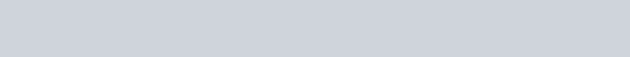The final marks in mathematics of 30 students are as follows:
Question:

The final marks in mathematics of 30 students are as follows:(i) Arrange these marks in the ascending order, 30 to 39 one group, 40 to 49 second group etc.

(ii) What is the highest score?

(iii) What is the lowest score?

(iv) What is the range?

(v) If 40 is the pass mark how many have failed?

(vi) How many have scored 75 or more?

(vii) Which observations between 50 and 60 have not actually appeared?

(viii) How many have scored less than 50?

Solution:

The given raw data can be arranged in an ascending order. The class intervals are $30-39,40-49, \ldots 100-109$. Then, take the raw data and place it in the appropriate class intervals.

(i) The marks can be arranged in an ascending order as shown below:

30 to $39 \rightarrow 37,39$

40 to $49 \rightarrow 44,48,48$

50 to $59 \rightarrow 50,52,53$

55, 56, 58, 58, 59

60 to $69 \rightarrow 60,60,60,61,62,64,67,68$

70 to $79 \rightarrow 70,75,77,78$

80 to $89 \rightarrow 84,88$

90 to $99 \rightarrow 90,98$

100 to $109 \rightarrow 100$

(ii) The highest score is 100.

(iii) The lowest score is 37.

(iv) The range is 100-37, i.e. 63 .

(v) If 40 is the passing mark, then the number of students who failed is 2 (i.e. 37, 39).

(vi) The number of students scoring 75 and above is 8 (i.e. 75, 77, 78, 84, 88, 90, 98, 100).

(vii) The marks 51, 54, and 57 do not actually appear between 50 and 60.

(viii) The number of students scoring less than 50 is 5 (i.e. 37, 39, 44, 48, 48).